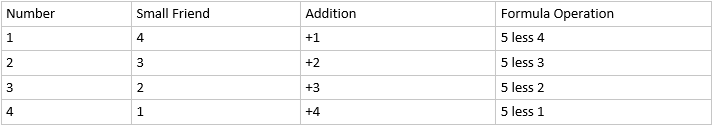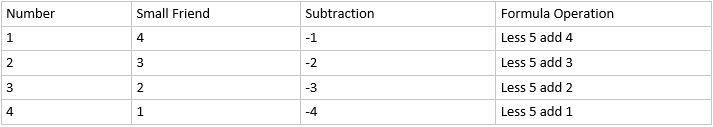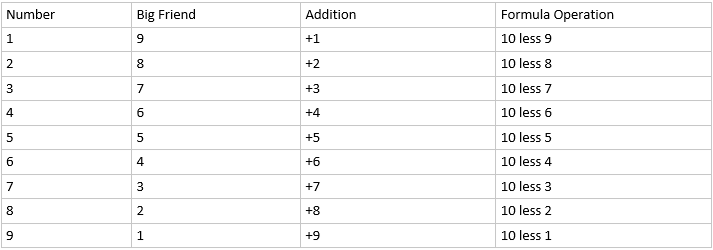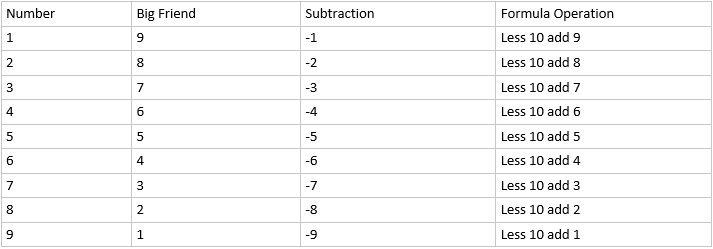# Abacus Formula: Big Friend & Small Friend Explained [Pdf]

Spread the word

Last Updated on February 17, 2021 by Editorial Team

Abacus is considered one of the important tools to promote mental math abilities in early learners. For those unaware, Abacus is a manual aid to calculating that consists of beads or disks that can be moved up and down on a series of sticks or strings within a usually wooden frame. It is considered one of the best ways to develop number sense.

Numbers are physically constructed and manipulated in Abacus. We already discussed different types of Abacus and their uses. Although not included in the regular math curriculum, but kids must try their hands on practicing various math operations with it once in a while.

If you just started learning the abacus, you must first learn the basics of the abacus. After getting your hands on the abacus and playing with it for a little while, its time to practice the basic operations (Addition & Subtraction) on it. In general practice, we take the help of the abacus formula to do addition and subtraction, especially when there are not enough beads in a column to add on Abacus. It involves using the concept of small friends and big friends for solving basic operations.

Look at the abacus formula sheet given below. You can also view/download pdf version by clicking this link.Abacus Formula Small Friend AdditionAbacus Formula Small Friend Substraction

In the case of Small friend, we take the bead of value 5 as the reference. To obtain the number from the addition and subtraction on abacus, we operate the reference 5 with the small friend. Here, we will explain it with examples of both.

READ :   What is Logical-mathematical Intelligence?: Importance, Activities, Examples and Its role in teaching

Let’s add 3+4,

1. Place 3 (3 lower beads of value 1) on abacus
2. Now, we have to add 4 but there are not enough beads left, so we will take the help of small friend as per the formula ‘add 5 less 1’.
3. We will add 5(1 upper bead on the same column) on abacus and remove 1 (1 lower bead on the same column )
4. We are left with (1 upper bead of 5, 2 lower bead of value 1). The solution is 5 + 2 = 7

Similarly, Let’s subtract 7 -4,

1. To make 7: Slide 2 lower beads of unit place and 1 upper bead of unit place (value 5).
2. To subtract 4, we have to take the help of big friend as there are not enough beads on the unit place. Use the formula ‘Less 5 add 1’.
3. Now remove 5 (slide down the upper bead of value 5) and add 1 (slide up the 1 lower bead of unit place)
4. We are left with (0 upper bead and 3 lower beads of unit’s place). The solution is 0 + 3 = 3, which is our answer.Abacus Formula Big Friend AdditionAbacus Formula Big Friend Subtraction

In the case of Big friend, we take the bead of value 10 as the reference. To obtain the number from the addition and subtraction on abacus, we operate reference 10 with the small friend. Here, we will explain it with examples of both.

Let’s add 8+9,

1. Place 8 (3 lower beads of value 1 and 1 upper bead of value 5) on abacus
2. Now, we have to add 9 but there are not enough beads left, so we will take the help of big friend as per the formula ‘add 10 less 1’.
3. We will add 10(1 lower bead on the adjacent left column) on abacus and remove 1 (1 lower bead on the same column )
4. We are left with (1 lower bead of value 10, 1 upper bead of 5, 2 lower bead of value 1). The solution is 10 + 5 + 2 = 17
READ :   Importance of spatial sense in kids and how to improve it?

Similarly, Let’s subtract 15 -7,

1. To make 15: Slide 1 lower bead of value 10 and 1 upper bead of unit place (value 5).
2. To subtract 7, we have to take the help of big friend as there are not enough beads in the unit place. Use the formula ‘Less 10 add 3’.
3. Now add 3 (slide up the 3 lower beads of unit place) and remove 10 (slide down the lower bead of value 10 columns).
4. We are left with (1 upper bead and 3 lower beads of unit’s place). The solution is 5 + 3 = 8, which is our answer.

`Want more fun printables? Check out PrintablesHub.com for worksheets, charts & game templates.`

Share the word

If you find this post helpful, then please help us spread the word. Share the post with your friends, family, and colleagues. Do not forget to subscribe to our updates. Any suggestions and recommendations are highly recommended. You can reach us through the contact form and we will get back at you shortly.

Spread the word